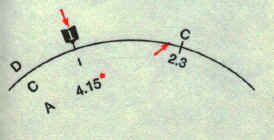Instruction for Use

New Circular Slide Rule

Multiplication

Of two given numbers
Rule:
Set 1 on scale C to multiply on scale D.
Against the multiplier on scale C read the product on scale D

 Example 1:1.8 x 2.5  = 4.5     Set on C to 1.8 on D.     Against 2.5 on C read 4.5 on D.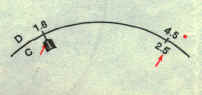Of three given numbers: Example 2: 3 x 4 x 5 = 60 Set 4 on Cl to 3 on D. Against 5 on C read 60 on D.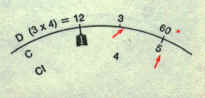Division

Of two given numbers:
Rule:
Set the divisor on C to the dividend on D.
Against the index on C read the quotient on D.

 Example 3: 850 : 25 = 34    Set 25 on C to 850 on D.    Against on C read 34 on 0.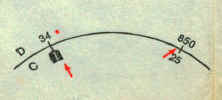Of three given numbers: Example 4: 850 : 25 : 8 4.25    Set 25 on C to 850 on D.    Against 8 on Cl read 4.25 on D.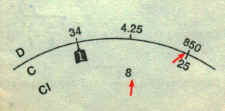Multiplication and Division

When multiplication and division are mixed together, you can of course do them one by one in continuation; but for the sake of speed there is a simpler method.
You may do both at once.

 Example 5:3 x 6 / 5 = 3.6    Set 5 on C to 3 on D.    Against 6 on C read 3.6 on D.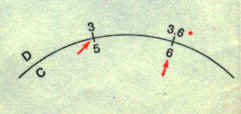Proportion

As an example of the mixture of multiplication and division, here are problems in proportion.

Proportion can be found by the use of reference scale method"with reference to C and D scale. This method is widely applied for conversion, indexes, proportional
division, percentage and also sale and purchase of commodities.

Rule:
In order to solve a:b = c:x,
Set a on C to b on D.
Against c on C read x on D.

Example 6:

 5:2.4 = 8:x   Ans: 3.84 Set 5 on C to 2.4 on D. Against 8 on C read 3.84 on D.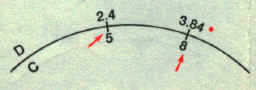Example 7 - Conversion
Fill in the following blanks, given 1 lb = 0.4536 kg.

 lbs 45 63 (50.7) (150) 180 kg (20.4) (28.6) 23 68 (81.6)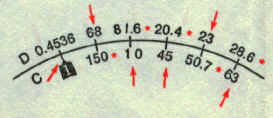For multiple calculations, scale should be fixed until the operation is finished.Above example indicating "lb" is set on C scale and "kg" on D scale with fixed relationship.

Example 8 - Percentage
Fill the blank columns in the following table

 A 350 (18.4) Make the total sum of parts: 350 + 450 + 500 + 600 = 1900.    Set 100 on C to 1900 on D.    Against 350, 450, 500, 600 on D,    Read the answers 18.4, 23.7, 26.3,31.6 on C. B 450 (23.7) C 500 (26.3) D 600 (31.6)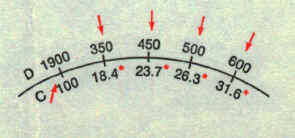Sum 1900 (100.)

 Example 9 - Sale and purchase:How much is 30 pcs. of a commodity at £ 15.00 per dozen? How many pcs. can be purchased at £ 4000? Ans: £ 37.50,            32 Pcs.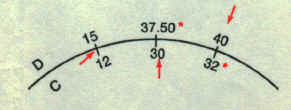Inverse proportion

With this circular slide rule, inverse proportion should always be done between D and Cl.

Example 10.There is a job 6 men complete in 14 days. How many days will it take for 8 men to finish the job?
Ans: 10.5 days.

 Set 6 on CI to 14 on 0. Against 8 on CI read 10.5 on D.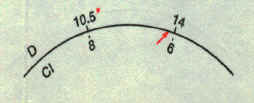Squares and square roots

To extract squares or square roots, answer may be secured with A and C scales in the reference relation.

Example 11:

 32 = 9      3.522 = 12.4 Ö 25 = 5 Ö (225)=15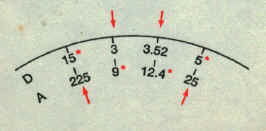Method of placing the decimal point for squares is the same as for that of multiplication.

In case of square roots, the given number is divided into several groups with two digits per group, counting from the decimal point in the direction of the first significant figure of a given number.

If the value in the top group is less than 10, the given number is set between 1 - 10 on A scale. If it is over 10, it is set between 10 - 100. Place the decimal point of the answer, taking one digit per group.

How to use Gauge mark c
There is a gauge mark "c at point 1.128. d . on D scale, which is used for relation of circle diameter and area, It is derived from the following formula:
area of circle a = (p/4) d2 d = diameter of circle
Changing the form, a = (Ö(pd/4)2 ) = (d/ Ö(4/p)2)
Denominator Ö(4/p) in parentheses corresponds to the value of c.

 Example 12: Find the area of a circle with diameter of 2.3 m. Ans 4.15 m2